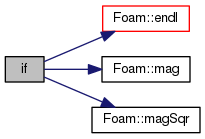The OpenFOAM Foundation
temperatureAndPressure.H File Reference

Accumulates values for temperature and pressure measurement, and calculates and outputs the average values at output times. Requires temperatureAndPressureVariables.H to be declared before the timeloop. More...

Go to the source code of this file.

## Functions

if (runTime.writeTime())

## Detailed Description

Accumulates values for temperature and pressure measurement, and calculates and outputs the average values at output times. Requires temperatureAndPressureVariables.H to be declared before the timeloop.

Original source file temperatureAndPressure.H

Definition in file temperatureAndPressure.H.

## ◆ if()

 if ( runTime. writeTime() )

Definition at line 48 of file temperatureAndPressure.H.

Here is the call graph for this function:## ◆ accumulatedTotalLinearMomentum

 accumulatedTotalLinearMomentum = singleStepTotalLinearMomentum

Definition at line 32 of file temperatureAndPressure.H.

Referenced by if().

## ◆ accumulatedTotalMass

 accumulatedTotalMass = singleStepTotalMass

Definition at line 34 of file temperatureAndPressure.H.

Referenced by if().

## ◆ accumulatedTotalLinearKE

 accumulatedTotalLinearKE = singleStepTotalLinearKE

Definition at line 36 of file temperatureAndPressure.H.

Referenced by if().

## ◆ accumulatedTotalAngularKE

 accumulatedTotalAngularKE = singleStepTotalAngularKE

Definition at line 38 of file temperatureAndPressure.H.

Referenced by if().

## ◆ accumulatedTotalPE

 accumulatedTotalPE = singleStepTotalPE

Definition at line 40 of file temperatureAndPressure.H.

Referenced by if().

## ◆ accumulatedTotalrDotfSum

 accumulatedTotalrDotfSum = singleStepTotalrDotf

Definition at line 42 of file temperatureAndPressure.H.

Referenced by if().

## ◆ accumulatedNMols

 accumulatedNMols = singleStepNMols

Definition at line 44 of file temperatureAndPressure.H.

Referenced by if().

## ◆ accumulatedDOFs

 accumulatedDOFs = singleStepDOFs

Definition at line 46 of file temperatureAndPressure.H.

Referenced by if().